Thank you for visiting nature.com. You are using a browser version with limited support for CSS. To obtain the best experience, we recommend you use a more up to date browser (or turn off compatibility mode in Internet Explorer). In the meantime, to ensure continued support, we are displaying the site without styles and JavaScript.

# Random focusing of tsunami waves

## Abstract

Tsunamis exhibit surprisingly strong height fluctuations. An in-depth understanding of the mechanisms that lead to these variations in wave height is a prerequisite for reliable tsunami forecasting. It is known, for example, that the presence of large underwater islands1 or the shape of the tsunami source2 can affect the wave heights. Here we show that the consecutive effect of even tiny fluctuations in the profile of the ocean floor (the bathymetry) can cause unexpectedly strong fluctuations in the wave height of tsunamis, with maxima several times higher than the average wave height. A novel approach combining stochastic caustic theory and shallow water wave dynamics allows us to determine the typical propagation distance at which the strongly focused waves appear. We demonstrate that owing to this mechanism the small errors present in bathymetry measurements can lead to drastic variations in predicted tsunami heights. Our results show that a precise knowledge of the ocean’s bathymetry is absolutely indispensable for reliable tsunami forecasts.

## Main

The devastating consequences of recent tsunami catastrophes have led to strongly increased efforts in tsunami research in the past decade, aiming at reliable predictions of tsunami properties3. Run-up measurements and detailed simulations of past tsunami events have shown pronounced fluctuations in the crest height of tsunamis (see, for example, http://nctr.pmel.noaa.gov/honshu20110311). Although in-detail numerical modelling of the wave propagation in the measured depth profile of the ocean floor captures many aspects of these fluctuations, a theoretical understanding of the focusing mechanisms that cause them will allow one to scrutinize and improve the assumptions made in these models. Figure 1 illustrates that bathymetry fluctuations of the order of only a few per cent of the ocean depth can indeed lead to tremendous variations in the wave height. As we will show below, this is related to the phenomenon of branched flow4, which has been observed in various contexts, in particular for linear waves propagating in a weak random potential or a weakly scattering random medium when the fluctuations of the medium are correlated on length scales longer than the wavelengths. Examples include the propagation of electron waves through high-mobility semiconductors4,5,6,7, sound propagation in the oceans8 and microwave transmission through a field of weak random scatterers9,10. It has also been related to the occurrence of freak waves in the oceans11,12,13. The origin of branched wave flows in these cases can best be understood in a corresponding ray picture, where one observes that consecutive weak scattering events in a random medium can lead to surprisingly strong focusing effects connected to the formation of random caustics6,13,14,15,16,17,18.Figure 1: Random focusing of a tsunami wave by the weakly fluctuating depth profile of the Indian Ocean.

The complexity of the geologic processes responsible for the depth profile of the ocean floor makes it natural to describe the bathymetry as a correlated random medium. As the stochastic ray equations describing tsunami propagation are very different, however, from the equations governing all the above-mentioned wave systems (there is an extra multiplicative noise term in the stochastic equations, see Methods), results obtained for the latter cannot be carried over and an appropriate theoretical description is required. In this Letter, we therefore present a theory for the branching of tsunami waves and the statistics of random focusing events. We derive the typical propagation distance for the occurrence of strongly enhanced wave heights and confirm our theoretical finding with extensive numerical experiments of ray and wave propagation in randomly generated bathymetries. We find that even bathymetry fluctuations as small as 2% of the ocean depth, when they are correlated on length scales larger than the wavelength, lead to focusing on length scales less than 2,000 km—that is, distances very relevant for tsunami propagation. To underline the importance of understanding these effects for tsunami forecasting, we will show by direct comparison of the wave propagation in very similar bathymetries that small uncertainties in the knowledge of the depth profile of the ocean can lead to vastly different branching patterns and thus to very different predictions of the tsunami propagation directions and heights.

Tsunami waves typically have wavelengths of several tens of kilometres19 and propagate across oceans that are only a few kilometres deep. Far from the coast, the amplitudes of such waves are of the order of one metre19. In consequence, tsunami waves are described well by the linearized shallow water wave equation (see Methods). The main quantity that influences the evolution of the waves is the bathymetry, which appears in the equation as a dimensionless, reduced bathymetry function given by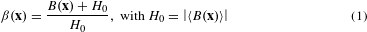as illustrated in Fig. 1c. Here the bathymetry B(x) < 0 is the actual depth measured from the sea level. The variance of the scaled bathymetry β(x), which we denote by 〈β2〉, is the crucial quantity that represents the fluctuation strength of the bathymetry.

As a first demonstration of the significance of branching for tsunami waves we study the propagation of shallow water waves over the measured depth profile of a region of the Indian Ocean (taken from the GEBCO database, http://www.gebco.net), shown in Fig. 1. This particular region was chosen because it is free of islands and very high underwater structures. It therefore allows us to clearly demonstrate the branching behaviour unobscured by strong scattering events. The waves, emitted from a point source, have a wavelength of 20 km and are thus on the lower end of the tsunami window. This wavelength was chosen for the sake of clarity and the relative smallness of the island-free region. However, our results rely neither on the specific wavelength nor on the shape of the source, as demonstrated in the Supplementary Figs 3 and 4. In the chosen region of the ocean the bathymetry varies only slightly, with a standard deviation of only 6.9% of the ocean depth, but strong fluctuations up to six times the average amplitude can nevertheless be observed in Fig. 1 owing to random focusing of the tsunami waves.

We now show how severe the impact of branching can be on the predictions of tsunami heights. Our knowledge of the depth profile of the ocean, as it is collected in the GEBCO database, is of course not perfect. The collected bathymetry data stem from different sources and are obtained by different methods, most prominently by echo sounding from ships and gravitational anomaly measurements from space. The uncertainty in the deep ocean bathymetry can easily be of the order of a few hundred metres20. In our example of the Indian Ocean, with an average ocean depth of 4 km, this corresponds to fluctuations of the order of several percent in the relative bathymetry. To see what kind of impact such uncertainties in the knowledge of the bathymetry could have, we added fluctuations with a variance of 4% to the data extracted from the database. As little is known of the statistical properties of the actual errors, we assumed a correlation function with a power-law decay, which is to be expected if the different sources of error have different typical length scales. We have checked a range of decay exponents γ, and also for comparison used Gaussian correlation functions, and in all cases observed results similar to the ones presented in Fig. 2, with γ = 1.2 (details are given in Methods, see equation (24)). We also used various values for the variance, going as low as 2%, and found the effect to be persistent. Figure 2a, b shows the bathymetry taken from the database and the bathymetry with added fluctuations, respectively. Already the density plots of the wave evolution in Fig. 2c and d show that these small additional fluctuations in the bathymetry change the branching pattern and propagation directions severely. Cuts along the green and the orange lines are shown in Fig. 2e, from which one can see that peaks in wave height of five to six times the average wave intensity appear at completely different positions for the two bathymetries.

Nevertheless, it seems possible to predict the average distance at which strong wave intensities first show up. With this aim and to unambiguously attribute the observed height fluctuations to the phenomenon of branched flow, we study the typical length scales at which these branched structures emerge and compare these to the typical length scale of caustic formation. In all of the previously studied cases of branched flows mentioned in the introduction, the wave dynamics is described well by a Schrödinger-type equation and the random potential leads to a correlated, additive noise in the corresponding stochastic dynamics of the rays. In these systems the typical length scale of branching has been studied theoretically and experimentally6,10,14,15,16. The linearized shallow water wave equations describing tsunami waves in the deep ocean, however, lead to multiplicative noise terms in the ray equations and earlier results on branched flows cannot be used to quantitatively link height fluctuations in the wave propagation to the branching mechanism described in ray models.

In Methods we therefore study the stochastic dynamics of tsunami rays analytically and derive the mean distance f that rays travel from the source before they hit the first caustic—that is, the typical propagation distance at which rays start to be strongly focused—as a function of the statistical parameters of the bathymetry. We find for small fluctuations in the bathymetry (that is, 〈β2 1) that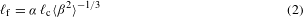where c is a characteristic or typical length scale on which the correlation function of the bathymetry decays, 〈β2〉 is the relative variance of the bathymetry and α is a proportionality constant that, for example, depends on the shape of the source. In spite of the different structure of the stochastic equations we still find the same expression as for Newtonian particles or optical rays.

To link the analytical result obtained for the rays to the propagation of tsunami waves, we perform extensive numerical simulations of both tsunami ray and wave propagation in different bathymetries, which we model as Gaussian correlated random fields (see Methods). We first verify the scaling of the distance to the first focusing in rays obtained in equation (2) by numerically determining the mean distance to a caustic along rays in many realizations of random bathymetries. Figure 3d shows excellent agreement between the analytical results and the numerical simulations in a broad range of variances of the bathymetry. We will now show that the focusing of the tsunami rays is indeed reflected in the wave fluctuations by comparing the characteristic length scales. We do this by analysing the variance of the wave intensity as a function of the propagation distance from the source10. This quantity shows a peak when the wave fluctuations are highest, which we attribute to the formation of caustics in the ray picture. To verify this we check whether the mean distance to the maximum of the variance of the wave intensity scales in the same way with the parameters of the bathymetry as the distance to the first caustic.Figure 3: Can we predict the mean distance to the strongest wave intensities?

More precisely, we normalize the variance and analyse the scintillation index of the wave flow, S(x) (ref. 10), defined by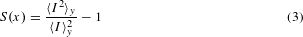where I(x, y) is the time-integrated intensity of the wave (see equation (21) in Methods), x the distance in the propagation direction and y the coordinate in the transverse direction. The index y in the variance and mean indicates that they are taken by averaging over the y-direction. Figure 3a shows the time-integrated intensity for one realization of a plane wave traversing a random potential. (For the sake of clarity we use plane waves instead of spherical waves. However, both have been shown to exhibit the same scaling behaviour6,16,21.) Figure 3b shows the associated scintillation index. We can see that the first peak of S(x) corresponds to the emergence of the first strong fluctuations.

To verify the scaling of the peak of the tsunami wave fluctuations, we averaged over 200 realization of the random bathymetry for each value of the variance of the bathymetry. The results are shown in Fig. 3c. The agreement between numerical simulation and the analytical scaling is again excellent over two orders of magnitude and thus confirms that our theory yields a very accurate forecast at which distance tsunami wave fluctuations are to be expected.

Our results show that small uncertainties in the knowledge of the bathymetry, when they are correlated on length scales comparable to or larger than the wavelength of the tsunami, can lead to large errors in the prediction of wave heights. Characterizing not only the magnitude but also the spatial correlations of uncertainties in the bathymetry model are thus fundamental prerequisites for assessing the reliability of tsunami predictions. Our work represents a general framework that can be used to account for the effect of branching and focusing in tsunami forecasts. Similar questions should be addressed about the impact of uncertainties of the source characteristics on the profile of branched tsunami flows.

## Methods

In deep ocean conditions, tsunami waves are described well by the linearized shallow water equation23,24: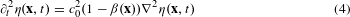where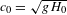is the celerity (that is, the phase velocity of the wave) with g Earth’s gravitational acceleration and H0 the absolute value of the average depth of the ocean.

The fractional surface elevation over the water surface at rest η(x, t) and the rescaled bathymetry β(x) are defined by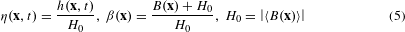with h(x, t) the actual surface elevation over the surface at rest and B(x) the actual bathymetry, measured from the sea level, as illustrated in Fig. 1c. We note that we neglect the Coriolis force, as it is very weak, and the curvature of the Earth, as its effect is negligible as long as the region of interest is well below the global scale24, which is well satisfied by the examples we treat here. But as both influences are smooth on very large scales even in larger simulations these corrections would affect only quantitative details of individual predictions and not the nature of the fluctuations we want to describe.

As focusing is an effect of the ray field associated with the waves25, we now turn to a ray description of the flow. Following the derivation by Shankar24, one obtains the ray equations for tsunami waves,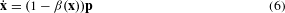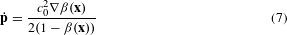The only assumption made during this derivation is that of the geometrical optics limit, which requires the wavelength to be shorter than the correlation length of the bathymetry. We have checked several regions of the Indian and Pacific oceans using the GEBCO database (http://www.gebco.net) and verified that the fluctuations in the bathymetry are typically correlated over length scales much longer than the typical length scales of tsunamis. We note that the first of these ray equations contains the multiplicative noise term β(x)p, which does not appear in Newtonian rays and which prevents us from directly transferring earlier results (for example, from ref. 16) to the random focusing of tsunami rays.

A crucial quantity describing the appearance of branches and thus of the strongly focused waves is the distance to the first focal regions, or caustics. As branched flow is due to random focusing, this distance is a stochastic quantity. We study equations (6) and (7) with random fields β(x) and calculate the mean distance to the first caustics. As we consider weakly fluctuating bathymetries, for the purpose of our analytical considerations we assume that the distance travelled in the main propagation direction is proportional to time, simplifying the problem to that of the ray dynamics in the transverse direction (paraxial approximation). Under these assumptions the correlated random bathymetry can be approximated by uncorrelated white noise terms14,15,16,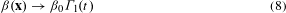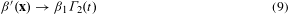with Γ1,2 defined by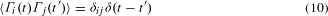The prefactors β0,1 now encode the properties of the random bathymetry and are given by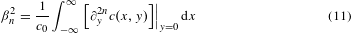where c(x, y) is the auto-correlation function of the bathymetry. The calculation yields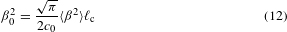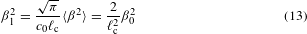Here we assumed a Gaussian correlation function. However, the Gaussian assumption is not necessary, and up to constant prefactors these expressions hold for a wide range of correlation functions, including power laws, and we expect them to lead to the same scaling behaviour16. We point out that, in contrast to the analytical results, all numerical simulations reported below have been performed using actual correlated bathymetries. With the above approximations, the ray equations become a set of stochastic differential equations,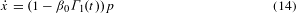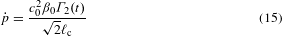where c denotes the correlation length of the bathymetry. A general solution for the distribution of the stochastic quantities x and p is not known, but its moments can be calculated and used for the estimation of the mean propagation distance to a focusing event in the following way: because the random medium is correlated, neighbouring rays will initially travel in the same direction. Only when they have travelled far enough in the main propagation direction such that they have traversed at least one correlation length in the transverse direction will rays start to intersect and focusing occur. A good estimate to the typical distance to a focusing event is thus the distance (or time in the paraxial approximation) travelled until the second moment of the distance covered in the transverse direction is equal to the correlation length squared of the bathymetry15. From the stochastic differential equations (14) and (15) we can derive the following Fokker–Planck equation,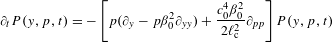This can be used to calculated the second moment that is needed in the calculation of the average distance to the first caustics. The closed set of equations for the second moments is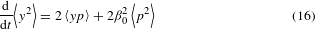The integration of this linear set of equations is straightforward and, using the initial conditions 〈p2〉 = 〈py〉 = 〈y2〉 = 0 at time t = 0, we obtain the second moment of y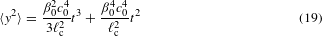and equating this with c2 yields, assuming β0 1,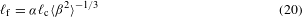where f denotes the distance to the first caustic and α is a proportionality factor that, for example, depends on the shape and characteristics of the source. Equations (19) and (20) relate the distance at which caustics occur, and thus the propagation distance at which large fluctuations in the wave height are to be expected to the parameters of the bathymetry, and are the main analytical result of this work. Interestingly, even though the linearized shallow water equations are very different from the Schrödinger equations, the scaling law is the same for both systems15,16.

The time-integrated intensity shown in the numerical plots we defined as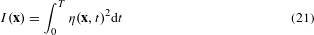where η(x, t) is the wave height and the tsunami is excited at time t = 0 and the upper integration limit t = T was chosen such that the wave has left the region of interest (that is, we neglect reflections, for example, from the coasts of continents, that are returning at longer times, which is reasonable in all shown examples). I(x) is a measure of the potential energy of the wave that has been propagating through x. Because we can to good accuracy assume equipartition of kinetic and potential energy of the propagating tsunami wave26, I(x) is an adequate measure of the total energy flux in x.

For the numerical integration of the ray equations, we used a fourth-order Runge–Kutta C++ solver. To simulate the linearized shallow water wave equations, we used a finite-difference, leapfrog scheme presented in ref. 27. The data shown in Fig. 3c was produced with a correlation-length-to-wavelength ratio of c/λ = 15. We verified that the same scaling holds for different ratios. In all simulations we use model sources that emit at a chosen wavelength, as described in ref. 28. The point source can be written as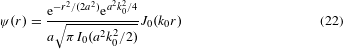where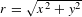, k0 is the magnitude of the wavevector, a is a scaling factor and I0 and J0 are Bessel functions. For plane waves, we use a very similar expression, given by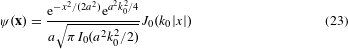More details can be found in ref. 28.

The correlations in the Gaussian random field used to create the additional fluctuations in the bathymetry for Fig. 2 have the form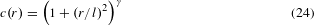with γ = −1.2 and l = 35 km. In Supplementary Fig. 3 we used l = 100 km. We checked that our results are not sensitive to the chosen values and obtained similar results in a wide range of parameter values.

## References

1. Berry, M. V. Focused tsunami waves. Proc. R. Soc. A 463, 3055–3071 (2007).

2. Kanoglu, U. et al. Focusing of long waves with finite crest over constant depth. Proc. R. Soc. A 469, 20130015 (2013).

3. Ward, S. N. & Day, S. Tsunami thoughts. Recorder J. Can. Soc. 30, 38–44 (2005).

4. Topinka, M. A. et al. Coherent branched flow in a two-dimensional electron gas. Nature 410, 183–186 (2001).

5. Aidala, K. E. et al. Imaging magnetic focusing of coherent electron waves. Nature Phys. 3, 464–468 (2007).

6. Maryenko, D. et al. How branching can change the conductance of ballistic semiconductor devices. Phys. Rev. B 85, 195329 (2012).

7. Liu, B. & Heller, E. J. Stability of branched flow from a quantum point contact. Phys. Rev. Lett. 111, 236804 (2013).

8. Wolfson, M. A. & Tomsovic, S. On the stability of long-range sound propagation through a structured ocean. J. Acoust. Soc. Am. 109, 2693–703 (2001).

9. Höhmann, R., Kuhl, U., Stöckmann, H.-J., Kaplan, L. & Heller, E. J. Freak waves in the linear regime: A microwave study. Phys. Rev. Lett. 104, 093901 (2010).

10. Barkhofen, S., Metzger, J. J., Fleischmann, R., Kuhl, U. & Stöckmann, H.-J. Experimental observation of a fundamental length scale of waves in random media. Phys. Rev. Lett. 111, 183902 (2013).

11. White, B. S. & Fornberg, B. On the chance of freak waves at sea. J. Fluid Mech. 355, 113–138 (1998).

12. Heller, E. Freak waves: Just bad luck, or avoidable? Europhys. News 36, 159–162 (2005).

13. Heller, E. J., Kaplan, L. & Dahlen, A. Refraction of a Gaussian seaway. J. Geophys. Res. 113, C09023 (2008).

14. Kulkarny, V. A. & White, B. Focusing of waves in turbulent inhomogeneous media. Phys. Fluids 25, 1770–1784 (1982).

15. Kaplan, L. Statistics of branched flow in a weak correlated random potential. Phys. Rev. Lett. 89, 184103 (2002).

16. Metzger, J. J., Fleischmann, R. & Geisel, T. Universal statistics of branched flows. Phys. Rev. Lett. 105, 020601 (2010).

17. Metzger, J. J., Fleischmann, R. & Geisel, T. Intensity fluctuations of waves in random media: What is the semiclassical limit? Phys. Rev. Lett. 111, 013901 (2013).

18. Metzger, J. J., Fleischmann, R. & Geisel, T. Statistics of extreme waves in random media. Phys. Rev. Lett. 112, 203903 (2014).

19. Ward, S. N. in The Encyclopedia of Physical Science and Technology (ed. Meyers, R. A.) 175–191 (Academic Press, 2002).

20. Sandwell, D., Gille, S. & Smith, W. Bathymetry from space: Oceanography, geophysics, and climate, geoscience professional services, Bethesda, Maryland (2002).

21. Metzger, J. J. Branched Flow and Caustics in Two-Dimensional Random Potentials and Magnetic Fields PhD thesis, Niedersächsische Staats-und Universitätsbibliothek Göttingen (2010).

22. Stöckli, R., Vermote, E., Saleous, N., Simmon, R. & Herring, D. The Blue Marble Next Generation—A True Color Earth Dataset Including Seasonal Dynamics From MODIS (NASA Earth Observatory, 2005).

23. George, D. L. Finite Volume Methods and Adaptive Refinement for Tsunami Propagation and Inundation PhD thesis, Univ. Washington (2006).

24. Shankar, R. in Tsunamis and Nonlinear Waves (ed. Kundu, A.) 263–272 (Springer, 2007).

25. Berry, M. V. & Upstill, C. Catastrophe optics: Morphologies of caustics and their diffraction patterns. Prog. Opt. 18, 257–346 (1980).

26. Dutykh, D. & Dias, F. Energy of tsunami waves generated by bottom motion. Proc. R. Soc. A 465, 725–744 (2009).

27. Lin, P. Numerical Modeling of Water Waves (Taylor and Francis, 2008).

28. Kramer, T., Heller, E. J. & Parrott, R. E. An efficient and accurate method to obtain the energy-dependent Green’s function for general potentials. J. Phys. Conf. Ser. 99, 012010 (2008).

## Acknowledgements

We thank E. Bodenschatz for fruitful discussions. This work has been supported by the DFG research group 760.

## Author information

Authors

### Contributions

The project was devised by J.J.M. and R.F., and developed jointly by all authors. All numerical work was performed by H.D. and J.J.M. The manuscript was written by all authors.

### Corresponding author

Correspondence to Theo Geisel.

## Ethics declarations

### Competing interests

The authors declare no competing financial interests.

## Supplementary information

### Supplementary Movie 1

Supplementary Movie (AVI 2927 kb)

## Rights and permissions

Reprints and Permissions

• ### Branched flows of flexural waves in non-uniform elastic plates

• Kevin Jose
• Neil Ferguson

Communications Physics (2022)

• ### Hydrodynamic aspects of tsunami wave motion: a review

• Shibam Manna
• Dipannita Maji

Ocean Dynamics (2021)

• ### Observation of branched flow of light

• Anatoly Patsyk
• Uri Sivan
• Miguel A. Bandres

Nature (2020)

• ### Effects of a natural disaster on mortality risks over the longer term

• Elizabeth Frankenberg
• Cecep Sumantri
• Duncan Thomas

Nature Sustainability (2020)

• ### Identifying the role of initial wave parameters on tsunami focusing

• Baran Aydın

Acta Geophysica (2018)

## Search